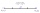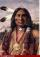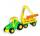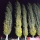# Midpoint 5

FM=3x-4, MG=5x-26, FG=? Point M is the midpoint of FG. Use the given information to find the missing measure or value.

Result

m =  58

#### Solution:Leave us a comment of example and its solution (i.e. if it is still somewhat unclear...):

Showing 0 comments:Be the first to comment!#### To solve this example are needed these knowledge from mathematics:

Do you have a linear equation or system of equations and looking for its solution? Or do you have quadratic equation? Do you want to convert length units?

## Next similar examples:

1. Find midpointFM=5y+13, MG=5-3y, FG=? M is the midpoint of FG. Use the given information to find the missing measure or value.
2. MG=7x-15,MG=7x-15, FG=33, x=? Point M is the midpoint of FG. Find unknown x.
3. Midpoint 6FM=8a+1, FG=42, a=? Point M is the midpoint of FG. Find unknown a.
4. GivenGiven 2x =0.125 find the value of x
5. Simple equationSolve for x: 3(x + 2) = x - 18
6. Simplify 2Simplify expression: 5ab-7+3ba-9
7. Unknown numberIdentify unknown number which 1/5 is 40 greater than one tenth of that number.
8. EquationSolve the equation: 1/2-2/8 = 1/10; Write the result as a decimal number.
9. EthnicityShare of ethnicity XY is 26%, which is 1/9 more than in the prewar period. What was the share of that ethnicity in the pre-war period?
10. CargoCar with cargo weight 7610 kg. Weight of car is 23% of the total weight of car and cargo. How heavy is cargo?
11. Unknown number 24f we add 20, we get 50% of its triple. What is this unknown number?
12. PoplarHow tall is a poplar by the river, if we know that 1/5 of its total height is a trunk, 1/10th of the height is the root and 35m from the trunk to the top of the poplar?
13. SeamstressThe seamstress cut the fabric into 3 parts. The first part was the eighth fabric, the second part was three-fifths of the fabric and the third part had a length of 66 cm. Calculate the original length of the fabric.
14. 1.5 divided1.5 divided by 1 = w divided by 4
15. One-thirdA one-third of unknown number is equal to five times as great as the difference of the same unknown number and number 28. Determine the unknown number.
16. Cleaning windowsCleaning company has to wash all the windows of the school. The first day washes one-sixth of the windows of the school, the next day three more windows than the first day and the remaining 18 windows washes on the third day. Calculate how many windows ha
17. Equation?